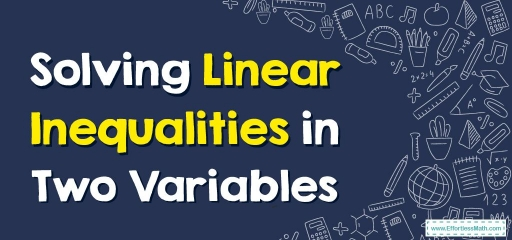# How to Solve Linear Inequalities in Two Variables?

Linear inequalities in two variables represent inequalities between two algebraic expressions in which two distinct variables are included. In the following guide, you will learn more about linear inequality in two variables and how to solve it.A linear inequality is almost identical to a linear equation, except that the equal sign is replaced by the symbol of inequality.

## A step-by-step guide tosolving linear inequalities in two variables

Linear inequalities in two variables represent an unequal relationship between two algebraic expressions that include two distinct variables. A linear inequality in two variables is formed when symbols other than equal to, such as greater than or less than are used to make a connection to expressions, and two variables are involved.

### How to solve linear inequalities with two variables?

The solution of linear inequalities in two variables is an ordered pair, which is true for the inequality statement. Let’s say that if $$Ax + By> C$$ is a linear inequality in which $$x$$ and $$y$$ are two variables, then an ordered pair $$(x, y)$$ that satisfies this expression would be the solution needed. Solving linear inequalities is like solving linear equations. The difference is in the symbol of inequality.

We solve linear inequalities in the same way as linear equations:

Step 1: Simplify the inequality on both sides, on $$LHS$$ as well as $$RHS$$ as per the rules of inequality.

Step 2: Once the value is obtained, we have:

• strict inequalities, in which the two sides of the inequalities cannot be equal to each other.
• non-strict inequalities, in which the two sides of the inequalities can also be equal.

Note: We saw that for every linear equation in two variables, there are infinite solutions. It may be clear to you now that we will have an infinite number of solutions to any linear inequality. All of these solutions constitute the solution set of a linear inequality.

### Solving Linear Inequalities in Two Variables – Example 1:

Determine whether $$(2, \frac{1}{5})$$ is a solution to $$2x+5y<10$$?

Solution:

Let’s put these values $$(2,\frac{1}{5})$$ in the given linear inequality, this gives:

$$2(2)+5(\frac{1}{5})<10$$

$$4+1<10$$

$$5<10$$, which holds true.

## Exercises forSolving Linear Inequalities in Two Variables

1. Check whether $$(3,-3)$$ is a solution of $$2x+3y<1$$?
2. Determine whether $$(-1,3)$$ is a solution of $$2x-6y>2$$?
1. $$\color{blue}{yes}$$
2. $$\color{blue}{no}$$

### What people say about "How to Solve Linear Inequalities in Two Variables? - Effortless Math: We Help Students Learn to LOVE Mathematics"?

No one replied yet.

X
30% OFF

Limited time only!

Save Over 30%

SAVE $5 It was$16.99 now it is \$11.99